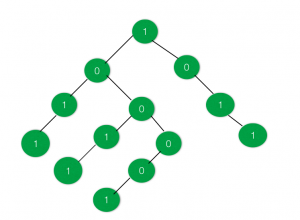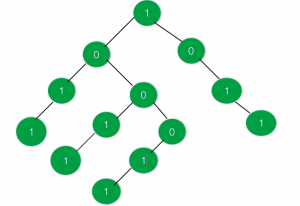Skip to content
Related Articles
Largest sub-tree having equal no of 1’s and 0’s
• Difficulty Level : Medium
• Last Updated : 02 Jun, 2021

Given a tree having every node’s value as either 0 or 1, the task is to find the maximum size of the sub-tree in the given tree that has equal number of 0’s and 1’s, if no such sub-tree exists then print -1.
Examples:

Input:Output: 6
Input:Output: -1

Approach:

1. Change all the nodes of the tree which are 0 to -1. Now the problem gets reduced to finding the maximum size of a sub-tree sum of whose nodes is 0.
2. Update all the nodes of the tree so that they represent the sum of all nodes in the sub-tree rooted at the current node.
3. Now find the size of the maximum sub-tree rooted at a node whose value is 0. If no such node is found then print -1

Below is the implementation of the above approach:

## C++

 `// C++ implementation of the approach``#include ``using` `namespace` `std;` `// To store the size of the maximum sub-tree``// with equal number of 0's and 1's``int` `maxSize = -1;` `// Represents a node of the tree``struct` `node {``    ``int` `data;``    ``struct` `node *right, *left;``};` `// To create a new node``struct` `node* newnode(``int` `key)``{``    ``struct` `node* temp = ``new` `node;``    ``temp->data = key;``    ``temp->right = NULL;``    ``temp->left = NULL;``    ``return` `temp;``}` `// Function to perform inorder traversal on``// the tree and print the nodes in that order``void` `inorder(``struct` `node* root)``{``    ``if` `(root == NULL)``        ``return``;``    ``inorder(root->left);``    ``cout << root->data << endl;``    ``inorder(root->right);``}` `// Function to return the maximum size of``// the sub-tree having equal number of 0's and 1's``int` `maxsize(``struct` `node* root)``{``    ``int` `a = 0, b = 0;``    ``if` `(root == NULL)``        ``return` `0;` `    ``// Max size in the right sub-tree``    ``a = maxsize(root->right);` `    ``// 1 is added for the parent``    ``a = a + 1;` `    ``// Max size in the left sub-tree``    ``b = maxsize(root->left);` `    ``// Total size of the tree``    ``// rooted at the current node``    ``a = b + a;` `    ``// If the current tree has equal``    ``// number of 0's and 1's``    ``if` `(root->data == 0)` `        ``// If the total size exceeds``        ``// the current max``        ``if` `(a >= maxSize)``            ``maxSize = a;` `    ``return` `a;``}` `// Function to update and return the sum``// of all the tree nodes rooted at``// the passed node``int` `sum_tree(``struct` `node* root)``{` `    ``if` `(root != NULL)` `        ``// If current node's value is 0``        ``// then update it to -1``        ``if` `(root->data == 0)``            ``root->data = -1;` `    ``int` `a = 0, b = 0;` `    ``// If left child exists``    ``if` `(root->left != NULL)``        ``a = sum_tree(root->left);` `    ``// If right child exists``    ``if` `(root->right != NULL)``        ``b = sum_tree(root->right);``    ``root->data += (a + b);` `    ``return` `root->data;``}` `// Driver code``int` `main()``{``    ``struct` `node* root = newnode(1);``    ``root->right = newnode(0);``    ``root->right->right = newnode(1);``    ``root->right->right->right = newnode(1);``    ``root->left = newnode(0);``    ``root->left->left = newnode(1);``    ``root->left->left->left = newnode(1);``    ``root->left->right = newnode(0);``    ``root->left->right->left = newnode(1);``    ``root->left->right->left->left = newnode(1);``    ``root->left->right->right = newnode(0);``    ``root->left->right->right->left = newnode(0);``    ``root->left->right->right->left->left = newnode(1);` `    ``sum_tree(root);` `    ``maxsize(root);` `    ``cout << maxSize;` `    ``return` `0;``}`

## Java

 `// Java implementation of the approach``class` `GFG``{` `// To store the size of the maximum sub-tree``// with equal number of 0's and 1's``static` `int` `maxSize = -``1``;` `// Represents a node of the tree``static` `class` `node``{``    ``int` `data;``    ``node right, left;``};` `// To create a new node``static` `node newnode(``int` `key)``{``    ``node temp = ``new` `node();``    ``temp.data = key;``    ``temp.right = ``null``;``    ``temp.left = ``null``;``    ``return` `temp;``}` `// Function to perform inorder traversal on``// the tree and print the nodes in that order``static` `void` `inorder(node root)``{``    ``if` `(root == ``null``)``        ``return``;``    ``inorder(root.left);``    ``System.out.print(root.data +``"\n"``);``    ``inorder(root.right);``}` `// Function to return the maximum size of``// the sub-tree having equal number of 0's and 1's``static` `int` `maxsize(node root)``{``    ``int` `a = ``0``, b = ``0``;``    ``if` `(root == ``null``)``        ``return` `0``;` `    ``// Max size in the right sub-tree``    ``a = maxsize(root.right);` `    ``// 1 is added for the parent``    ``a = a + ``1``;` `    ``// Max size in the left sub-tree``    ``b = maxsize(root.left);` `    ``// Total size of the tree``    ``// rooted at the current node``    ``a = b + a;` `    ``// If the current tree has equal``    ``// number of 0's and 1's``    ``if` `(root.data == ``0``)` `        ``// If the total size exceeds``        ``// the current max``        ``if` `(a >= maxSize)``            ``maxSize = a;` `    ``return` `a;``}` `// Function to update and return the sum``// of all the tree nodes rooted at``// the passed node``static` `int` `sum_tree(node root)``{` `    ``if` `(root != ``null``)` `        ``// If current node's value is 0``        ``// then update it to -1``        ``if` `(root.data == ``0``)``            ``root.data = -``1``;` `    ``int` `a = ``0``, b = ``0``;` `    ``// If left child exists``    ``if` `(root.left != ``null``)``        ``a = sum_tree(root.left);` `    ``// If right child exists``    ``if` `(root.right != ``null``)``        ``b = sum_tree(root.right);``    ``root.data += (a + b);` `    ``return` `root.data;``}` `// Driver code``public` `static` `void` `main(String[] args)``{``    ``node root = newnode(``1``);``    ``root.right = newnode(``0``);``    ``root.right.right = newnode(``1``);``    ``root.right.right.right = newnode(``1``);``    ``root.left = newnode(``0``);``    ``root.left.left = newnode(``1``);``    ``root.left.left.left = newnode(``1``);``    ``root.left.right = newnode(``0``);``    ``root.left.right.left = newnode(``1``);``    ``root.left.right.left.left = newnode(``1``);``    ``root.left.right.right = newnode(``0``);``    ``root.left.right.right.left = newnode(``0``);``    ``root.left.right.right.left.left = newnode(``1``);` `    ``sum_tree(root);` `    ``maxsize(root);` `    ``System.out.print(maxSize);``}``}` `// This code is contributed by PrinciRaj1992`

## C#

 `// C# implementation of the approach``using` `System;` `class` `GFG``{` `// To store the size of the maximum sub-tree``// with equal number of 0's and 1's``static` `int` `maxSize = -1;` `// Represents a node of the tree``public` `class` `node``{``    ``public` `int` `data;``    ``public` `node right, left;``};` `// To create a new node``static` `node newnode(``int` `key)``{``    ``node temp = ``new` `node();``    ``temp.data = key;``    ``temp.right = ``null``;``    ``temp.left = ``null``;``    ``return` `temp;``}` `// Function to perform inorder traversal on``// the tree and print the nodes in that order``static` `void` `inorder(node root)``{``    ``if` `(root == ``null``)``        ``return``;``    ``inorder(root.left);``    ``Console.Write(root.data +``"\n"``);``    ``inorder(root.right);``}` `// Function to return the maximum size of``// the sub-tree having equal number of 0's and 1's``static` `int` `maxsize(node root)``{``    ``int` `a = 0, b = 0;``    ``if` `(root == ``null``)``        ``return` `0;` `    ``// Max size in the right sub-tree``    ``a = maxsize(root.right);` `    ``// 1 is added for the parent``    ``a = a + 1;` `    ``// Max size in the left sub-tree``    ``b = maxsize(root.left);` `    ``// Total size of the tree``    ``// rooted at the current node``    ``a = b + a;` `    ``// If the current tree has equal``    ``// number of 0's and 1's``    ``if` `(root.data == 0)` `        ``// If the total size exceeds``        ``// the current max``        ``if` `(a >= maxSize)``            ``maxSize = a;` `    ``return` `a;``}` `// Function to update and return the sum``// of all the tree nodes rooted at``// the passed node``static` `int` `sum_tree(node root)``{` `    ``if` `(root != ``null``)` `        ``// If current node's value is 0``        ``// then update it to -1``        ``if` `(root.data == 0)``            ``root.data = -1;` `    ``int` `a = 0, b = 0;` `    ``// If left child exists``    ``if` `(root.left != ``null``)``        ``a = sum_tree(root.left);` `    ``// If right child exists``    ``if` `(root.right != ``null``)``        ``b = sum_tree(root.right);``    ``root.data += (a + b);` `    ``return` `root.data;``}` `// Driver code``public` `static` `void` `Main(String[] args)``{``    ``node root = newnode(1);``    ``root.right = newnode(0);``    ``root.right.right = newnode(1);``    ``root.right.right.right = newnode(1);``    ``root.left = newnode(0);``    ``root.left.left = newnode(1);``    ``root.left.left.left = newnode(1);``    ``root.left.right = newnode(0);``    ``root.left.right.left = newnode(1);``    ``root.left.right.left.left = newnode(1);``    ``root.left.right.right = newnode(0);``    ``root.left.right.right.left = newnode(0);``    ``root.left.right.right.left.left = newnode(1);` `    ``sum_tree(root);` `    ``maxsize(root);` `    ``Console.Write(maxSize);``}``}` `// This code is contributed by Rajput-Ji`

## Javascript

 ``
Output:
`6`

Attention reader! Don’t stop learning now. Get hold of all the important DSA concepts with the DSA Self Paced Course at a student-friendly price and become industry ready.  To complete your preparation from learning a language to DS Algo and many more,  please refer Complete Interview Preparation Course.

In case you wish to attend live classes with industry experts, please refer DSA Live Classes

My Personal Notes arrow_drop_up